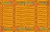Crownnote is where music fans share their favorite songs.

Chart

# Morneau's 2019 Year End List

It's the end of 2019, the end of the decade as we know it, and it's time for another year-end list. A year dominated by Cage the Elephant, Local Natives, Cayucas, Foals, Silversun Pickups, etc. And impressive comebacks from Meg Myers, Bad Suns, COIN, Saint Motel, and so on, so on.

Let the year-end list begin!

1. Number
1
2. Number
2
3. Number
3
4. Number
4
5. Number
5
6. Number
6
7. Number
7
8. Number
8
9. Number
9
10. Number
10
11. Number
11
12. Number
12
13. Number
13
14. Number
14
15. Number
15
16. Number
16
17. Number
17
18. Number
18
19. Number
19
20. Number
20
21. Number
21
22. Number
22
23. Number
23
24. Number
24
25. Number
25
26. Number
26
27. Number
27
28. Number
28
29. Number
29
30. Number
30
31. Number
31
32. Number
32
33. Number
33
34. Number
34
35. Number
35
36. Number
36
37. Number
37
38. Number
38

39. Number
39
40. Number
40
41. Number
41
42. Number
42
43. Number
43
44. Number
44
45. Number
45
46. Number
46
47. Number
47
48. Number
48
49. Number
49
50. Number
50
51. Number
51
52. Number
52
53. Number
53
54. Number
54
55. Number
55
56. Number
56
57. Number
57
58. Number
58
59. Number
59
60. Number
60
61. Number
61
62. Number
62
63. Number
63
64. Number
64
65. Number
65
66. Number
66
67. Number
67
68. Number
68
69. Number
69
70. Number
70
71. Number
71
72. Number
72
73. Number
73
74. Number
74
75. Number
75
76. Number
76
77. Number
77
78. Number
78

## Boys Grow Up

Artificial Pleasure featuring JYLDA

79. Number
79
80. Number
80
81. Number
81
82. Number
82
83. Number
83
84. Number
84
85. Number
85
86. Number
86
87. Number
87
88. Number
88
89. Number
89
90. Number
90
91. Number
91
92. Number
92
93. Number
93
94. Number
94
95. Number
95
96. Number
96
97. Number
97
98. Number
98
99. Number
99
100. Number
100
101. Dropouts
1. Number
4 Dropout
Peak
4
Weeks
7
2. Number
8 Dropout
Peak
8
Weeks
7
3. Number
14 Dropout
Peak
14
Weeks
10
4. Number
16 Dropout
Peak
16
Weeks
6
5. Number
17 Dropout
Peak
17
Weeks
12
6. Number
18 Dropout
Peak
18
Weeks
6
7. Number
21 Dropout
Peak
21
Weeks
9
8. Number
22 Dropout
Peak
22
Weeks
6
9. Number
23 Dropout
Peak
23
Weeks
9
10. Number
26 Dropout
Peak
26
Weeks
5
11. Number
27 Dropout
Peak
22
Weeks
9
12. Number
28 Dropout
Peak
28
Weeks
5
13. Number
29 Dropout
Peak
29
Weeks
8
14. Number
30 Dropout
Peak
30
Weeks
10
15. Number
31 Dropout
Peak
31
Weeks
6
16. Number
32 Dropout
Peak
24
Weeks
13
17. Number
33 Dropout
Peak
33
Weeks
5
18. Number
34 Dropout
Peak
34
Weeks
6
19. Number
35 Dropout
Peak
35
Weeks
9
20. Number
36 Dropout
Peak
36
Weeks
4
21. Number
37 Dropout
Peak
37
Weeks
3
22. Number
38 Dropout
Peak
38
Weeks
4
23. Number
40 Dropout
Peak
40
Weeks
3
24. Number
41 Dropout
Peak
41
Weeks
2
25. Number
42 Dropout
Peak
42
Weeks
2
26. Number
43 Dropout
Peak
43
Weeks
1
27. Number
44 Dropout
Peak
44
Weeks
3
28. Number
46 Dropout
Peak
46
Weeks
1
29. Number
47 Dropout
Peak
45
Weeks
4
30. Number
48 Dropout
Peak
32
Weeks
14
31. Number
49 Dropout
Peak
49
Weeks
1
32. Number
50 Dropout
Peak
50
Weeks
1

A chart byMorneauMispelled
Date
Songs
100
No points